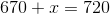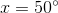## Example Questions

### Example Question #1 : How To Find An Angle In A Hexagon

The sum of all the angles inside of a regular hexagon is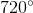. Determine the value of one angle.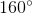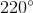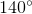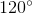Explanation:

In a regular hexagon, all of the sides are the same length, and all of the angles are equivalent. The problem tells us that all of the angles inside the hexagon sum to. To find the value of one angle, we must divideby, since there areangles inside of a hexagon.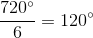### Example Question #13 : Hexagons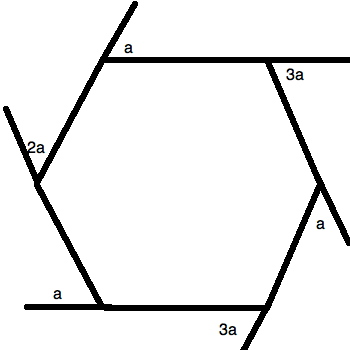All of the angles marked are exterior angles.

What is the value ofin degrees? Round to the nearest hundredth.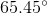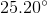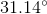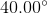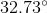Explanation:

There are two key things for a question like this. The first is to know that a polygon has a total degree measure of: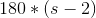, whereis the number of sides.

Therefore, a hexagon like this one has: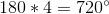.

Next, you should remember that all of the exterior angles listed are supplementary to their correlative interior angles. This lets you draw the following figure: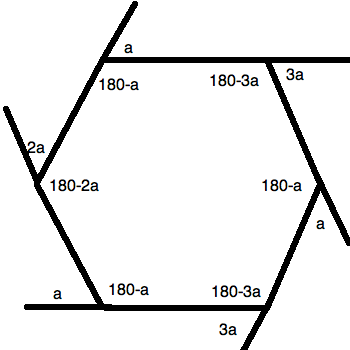Now, you just have to manage your algebra well. You must sum up all of the interior angles and set them equal to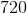. Since there areangles, you know that the numeric portion will be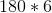or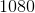. Thus, you can write: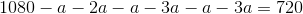Simplify and solve for: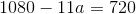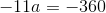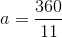This is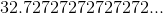or.

### Example Question #14 : Hexagons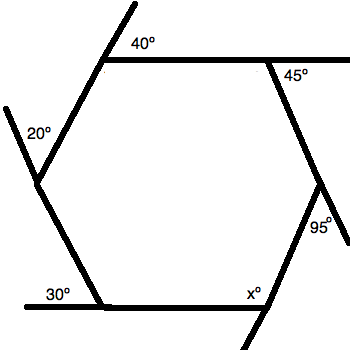The figure above is a hexagon.  All of the angles listed (except the interior one) are exterior angles to the hexagon's interior angles.

What is the value of?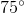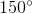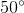Explanation:

There are two key things for a question like this. The first is to know that a polygon has a total degree measure of:, whereis the number of sides.

Therefore, a hexagon like this one has:.

Next, you should remember that all of the exterior angles listed are supplementary to their correlative interior angles.  This lets you draw the following figure: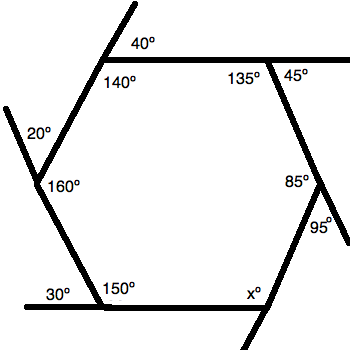Now, you just have to manage your algebra well. You must sum up all of the interior angles and set them equal to. Thus, you can write: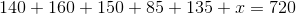Solve for: